﻿ Electronic and Structural Properties of Ca<SUB>x</SUB>Cd<SUB>1</SUB><SUB>−</SUB><SUB>x</SUB>F<SUB>2</SUB> and Ca<SUB>x</SUB>Mg<SUB>1</SUB><SUB>−</SUB><SUB>x</SUB>F<SUB>2</SUB>American Journal of Materials Science

p-ISSN: 2162-9382    e-ISSN: 2162-8424

2022;  12(1): 7-17

doi:10.5923/j.materials.20221201.02

Received: Oct. 30, 2021; Accepted: Nov. 29, 2021; Published: Mar. 15, 2022### Electronic and Structural Properties of CaxCd1−xF2 and CaxMg1−xF2

A. B. Olanipekun1, A. C. Obaze2

1Department of Pure and Applied Physics, Caleb University, Imota, Lagos State, Nigeria

2Department of Physics, Olabisi Onabanjo University, Ago-Iwoye, Ogun State, Nigeria

Correspondence to: A. B. Olanipekun, Department of Pure and Applied Physics, Caleb University, Imota, Lagos State, Nigeria.
 Email: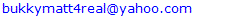Abstract

Using ab-initio calculations, band gap opening, elastic, electronic and structural properties of CaxCd1-xF2 and CaxMg1-xF2 alloys with 25%, 50% and 75% of Ca are studied. The calculations are performed using the pseudopotential and the plane wave basis sets while the exchange-correlation functional used is the Perdew and Wang's Generalized Gradient Approximation (GGA). First, the lattice constants a and bulk modulus B, of CaF2, CdF2, MgF2 and their ternary alloys were calculated. The lattice constants and bulk moduli of the binary compounds compare well with experiments. From the estimated bulk modulus values, CaxCd1-xF2 appears more compressible than CaxMg1-xF2 at the corresponding concentrations. In addition to PW calculation of the band gap, G0W0 method is employed here. The band structures and the density of states of the mixed divalent metal fluorides are presented. In the alloys, the valence bands are dominated by s-orbitals of Fluorine, the cations and the p states of F atoms. The conduction bands contain the contributions from the p and d states of the cations.

Keywords: Lattice constant, Bulk modulus, Band gap, Band structure, Density of states

Cite this paper: A. B. Olanipekun, A. C. Obaze, Electronic and Structural Properties of CaxCd1xF2 and CaxMg1xF2, American Journal of Materials Science, Vol. 12 No. 1, 2022, pp. 7-17. doi: 10.5923/j.materials.20221201.02.

### 1. Introduction

The divalent metal fluorides have wide band gaps and low refractive indices relative to other halides. They are commonly used in optical coatings and used as transmissive components in the deep ultraviolet . CaF2 and CdF2 are used in hetero-epitaxial fluoride structures which are used as materials for quantum well devices such as resonant tunneling devices on Si substrates . The MgF2 crystal has many technological applications, such as its use in the light polarizers and anti-reflective coatings [3,4]. Considering their high technological importance, the electronic properties of divalent metal fluorides have been calculated by different authors from first principles [5-10]. The electronic and structural properties of MgF2 have been calculated using; the tight binding method , a combined tight binding and pseudopotential method , coupling quantum-mechanical ab initio perturbed ion calculations with a quasi-harmonic Debye model , the self-consistent tight binding linear muffin tin orbital method (TB-LMTO) , ab initio code Crystal-2003 and the hybrid exchange-correlation B3PW functional , ab initio method based on density functional theory and norm-conserving pseudo potentials .
The ground state properties and electronic band structures of CaF2, SrF2 and CdF2 have been calculated using; the shell model in the pair potential approximation , the localized Gaussian-type basis set , plane waves expansion of the wave functions by means of density functional theory within the local density approximation for the exchange correlation energy , density functional theory and many-body perturbation theory . The electronic structure, the densities of states and lattice dynamical properties of CdF2 crystals have been calculated using the full-potential linear muffin-tin orbital method in the local density approximation  and ab initio calculations, based on density functional theory and norm-conserving pseudopotentials .
The high-pressure, phase transition sequence for MgF2 is rutile-CaCl2 - αPbO2 - PdF2 (modified fluorite) - αPbCl2, with an overall increase in the coordination number of Mg2 from 6 to 9 .
There are publications on the epitaxial growth of mixed divalent metal fluoride alloys, the fabrication of RTDs containing the fluoride alloy heterostructures and their electrical properties [24-27]. The mixed divalent metal fluorides (CaxCd1−xF2 and CaxMg1−xF2) have smooth surface morphology and good crystalline quality compared to the pure divalent metal fluorides [24,25,28].
Izumi and his co-workers  demonstrated that CaxMg1−xF2 and CaxCd1−xF2 are effective to overcome the problems of pin-hole effects in CaF2 and strong chemical reaction of CdF2 in resonant tunneling diodes composed of CaF2/CdF2/CaF2 on Si.
Inspite of the technological importance of the ternary alloys, a number of fundamental physical properties (electronic and structural properties) of these alloys are still unknown. A knowledge of these properties could help improve their performances and possibly propose new areas of applications.

### 2. Computational Method

Ab initio DFT calculation [30,31] is used to study the electronic and structural properties of CaxCd1−xF2 and CaxMg1−xF2. Generalised Gradient Approximation (GGA) with parametrization of Perdew and Wang is used for the exchange-correlation potential . The pseudopotentials generated with scalar relativistic calculation by Gonze X. et al.  for Ca, Cd, Mg and F are used. The configuration 3s2,3p6,4s2 of Ca, 4d10,5s2 of Cd, 3s2 of Mg and 2s2,2p5 of F are taken as valence states. The kinetic energy cut-off of 60Ryd and the Monkhorst and Pack scheme  for BZ integration are used. After thorough investigation, we obtained 6x6x6 k points for the total energy calculation of the fluorite crystals while 12x12x6 k-points is used for the rutile MgF2. The calculated total energy of the crystals converged to less than 1mRyd after seven iterations. The lattice constants and bulk moduli are calculated by fitting the total energy to the Murnaghan's equation of state .
The structural properties of the binary compounds CaF2 and CdF2 in the fluorite phase and MgF2 in the rutile phase using GGA scheme are calculated. The ternary alloys in cubic fluorite structures are modelled at some selected compositions of x and are described by periodically repeated supercells.
Density Functional Theory calculations yield good results for structural properties, but not for electronic properties. The electronic band gaps are underestimated. This is because DFT Kohn-Sham eigenvalues are treated as single-particle excitation energies. GW accurately predicts band gap based on the self-consistent quasiparticle energies . The huge computational cost of GW led to the discovery of G0W0 where the Green’s function (G) and screened dielectric potential (W) are calculated once from the KS density. Calculations within this approach have been applied to many materials. They generate band gaps that are comparable with the experimental values, although for some materials, higher deviations are noticed [37-39]. G0W0 approach which uses DFT-GGA orbitals and eigenvalues as starting point is used here.

### 3. Results and Discussion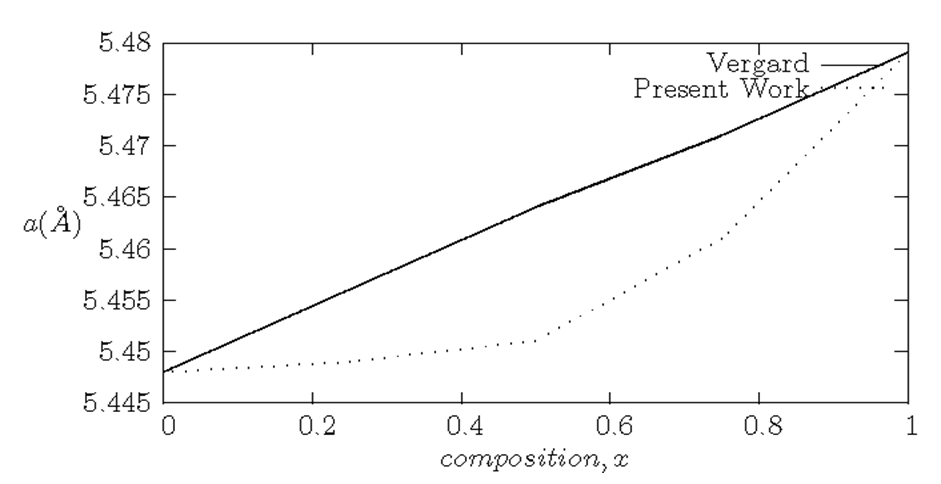Figure 1. Calculated lattice constants a at different compositions in CaxCd1-xF2; showing decreasing a as Ca increases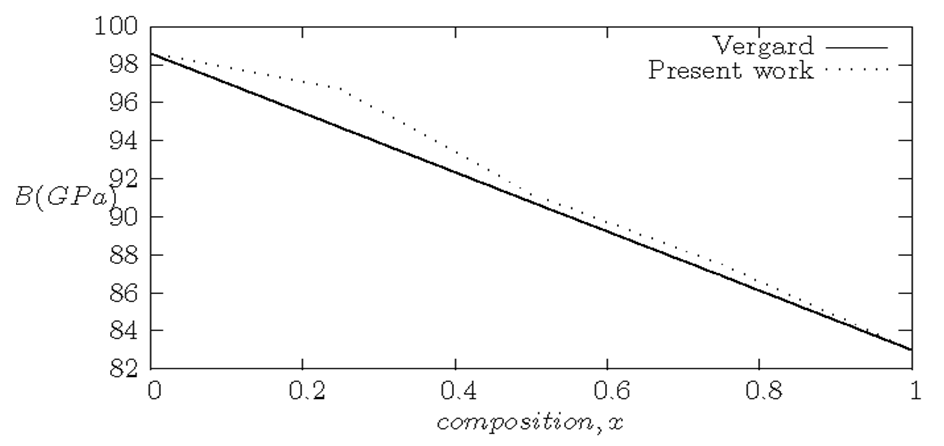Figure 2. Variation of bulk modulus with composition in CaxCd1-xF2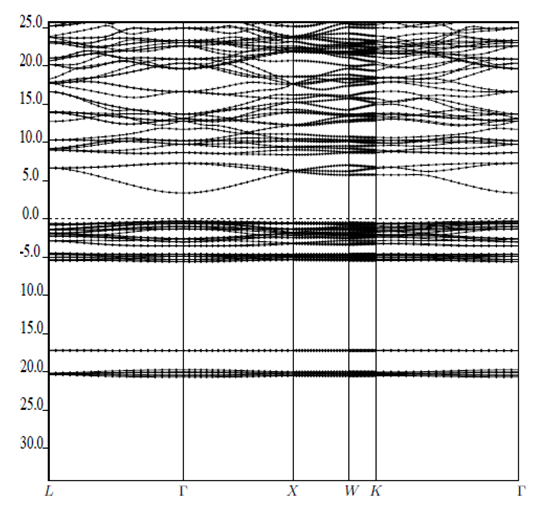Figure 3. Electronic band structure of Ca0.25Cd0.75F2 alloy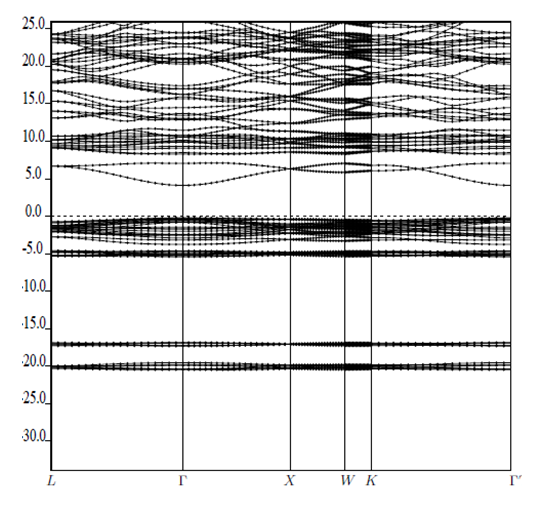Figure 4. Electronic band structure of Ca0.50Cd0.50F2 alloy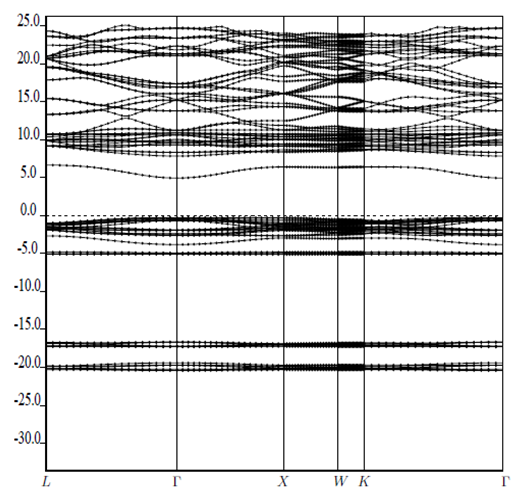Figure 5. Electronic band structure of Ca0.75Cd0.25F2 alloy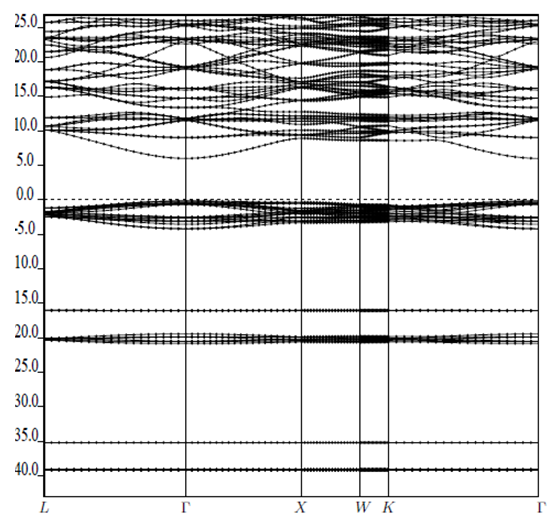Figure 6. Electronic band structure of Ca0.25Mg0.75F2 alloy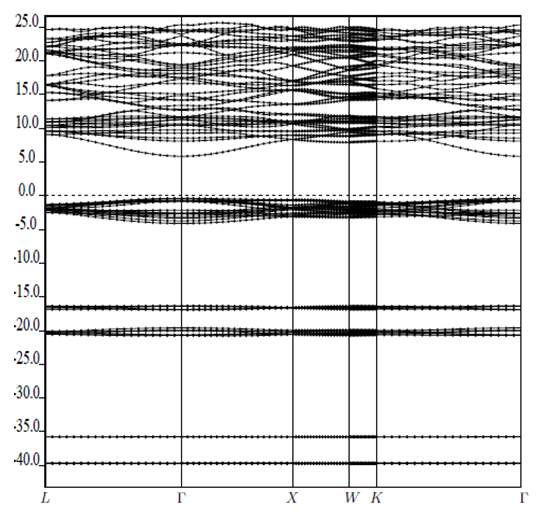Figure 7. Electronic band structure of Ca0.50Mg0.50F2 alloy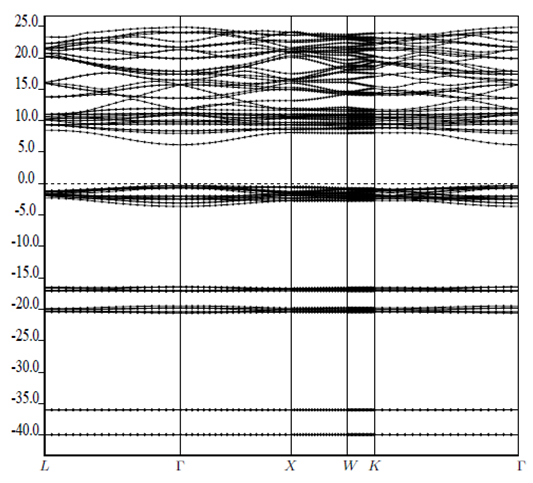Figure 8. Electronic band structure of Ca0.75Mg0.25F2 alloy
For Figures 3-8, vertical axis represents Energy (eV) while horizontal axis represents wave vector, k.
The structural properties of the binary compounds CaF2 and CdF2 in the fluorite phase and MgF2 in the rutile phase using GGA are calculated. Then the alloys in cubic fluorite structures were studied at selected compositions of x = 0.25, 0.50 and 0.75. The alloys are described in terms of supercells method. The structural optimization is done by minimizing the total energy with respect to the lattice constants and the atomic positions. The equilibrium structural properties were obtained for the binary compounds and their alloys. Our calculated values for the equilibrium lattice constant, a and bulk modulus, B for the binary compounds and their alloys are given in the Table 1 and Table 2 together with available theoretical and experimental data. The values for the lattice parameters a and the bulk modulus B for the binary compounds are in very good agreement with the experimental ones. The lattice parameter of the CaxCd1−xF2 and CaxMg1−xF2 ternary alloys decreases with composition, x while the bulk modulus increases with x, except at x=0 for CaxMg1−xF2. In CaxMg1−xF2 ternary alloys, though CaF2 has a fluorite structure type but MgF2 is a rutile in which the cell parameters are given as a = b ≠ c. And so, a and c are calculated as the cell parameters. It has already been shown that CaxMg1−xF2 has a cubic fluorite structure over a wide range of composition, i.e. 0.2xSn1−xO2 in which CeO2 has a fluorite structure while SnO2 is a rutile but Ce0.50Sn0.50O2 and Ce0.75Sn0.25O2 are fluorite . The calculated bulk modulus revealed that CaxMg1−xF2 is harder than CaxCd1−xF2.
 Table 1. Calculated Lattice Parameters, a(Å) of the Ternary Alloys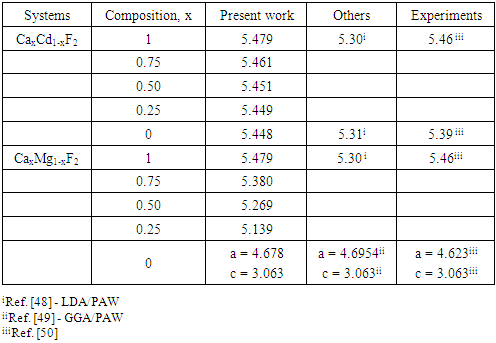Table 2. Calculated Bulk modulus, B(GPa) of the Ternary AlloysUsing Vegard's Law , effect of combining two binary compounds on structural properties (lattice constants and bulk modulus) of alloys can be explained.
A linear relation between the structural properties of the alloy and the composition x is possible by taking the weighted average of the corresponding structural properties of the binary compounds. However, the linear behaviour is not followed by many alloys and a general form is expressed by a semi-empirical quadratic relationship [43-44]. The deviation is measured by a bowing parameter b.
Figure 1 shows calculated lattice constants at different compositions of CaxCd1-xF2 alloys. We observed that the lattice parameter (a) of the ternary alloy decreases with an increase in Ca concentration. We compared calculated lattice parameters with those obtained from Vegard's Law at different x. From the results, there is a marginal downward bowing of 0.047Å due to smaller size of Calcium (Ca) atom (than that of Cadmium, Cd).
Figure 2 shows the variation of the bulk modulus as a function of the composition x in CaxCd1-xF2, the bulk modulus increases with x. A significant deviation from the linear concentration dependence occurs with upward bowing of -5.17GPa. This deviation may be due to the mismatches of the bulk modulus in the binary compounds.
In Table 3, the energy band-gaps are calculated at the principal symmetry points, alongside the experimental and theoretical data in the literature. The deviation is due to the fact that the density functional theory (DFT) underestimates the bandgap energy. However, DFT provides electronic band structures which are in good agreement with experiments qualitatively [45-47]. G0W0 gives good estimation of band gap for CaF2 and MgF2 but for CdF2, there is high discrepancy. G0W0 calculations of the band gap show a better agreement with experiment than PW calculations. The band gap of the CaxCd1−xF2 ternary alloys decreases with Ca contents. Their calculated band gap energies show that all the alloys are insulators which can be used as photodetectors working in the ultraviolet region of light.
 Table 3. Calculated Band gap energy, Eg(eV) of the Ternary Alloys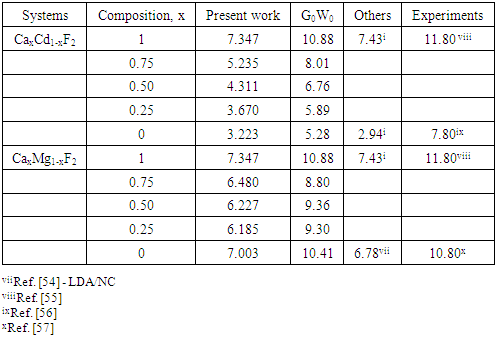The calculated electronic structure along high symmetry directions for ternary alloys CaxCd1−xF2 and CaxMg1−xF2 are displayed in Figures 2-8 for the first time in the literature. In this figures, the Fermi level EF is located at 0eV. The valence band maximum and the conduction band minimum occur at the Γ point indicating that these ternary materials have direct band gaps.
Figures 9, 10, 11 and 12 show the partial density of states of Ca, F, Mg and Cd respectively. The red line represents s-orbitals, green line represents p-orbitals and blue line represents d-orbitals. Ca, Mg and Cd contain s, p and d orbitals while F contains s and p orbitals. The partial density of states of the basic elements of the ternary alloys are used to explain the total density of states of the alloys.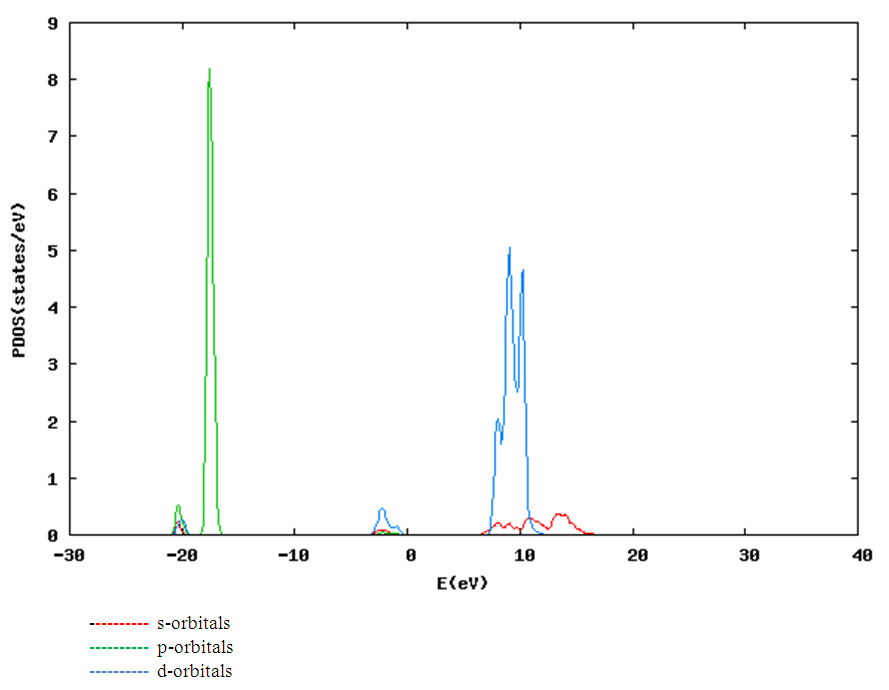Figure 9. Partial density of states (PDOS) of Calcium (Ca)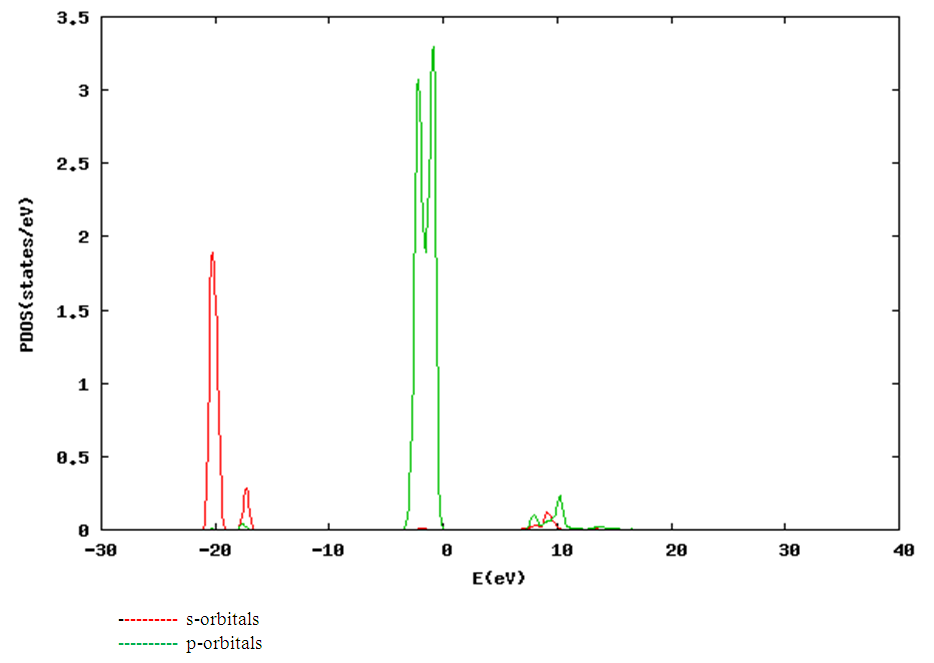Figure 10. Partial density of states (PDOS) of Fluorine (F)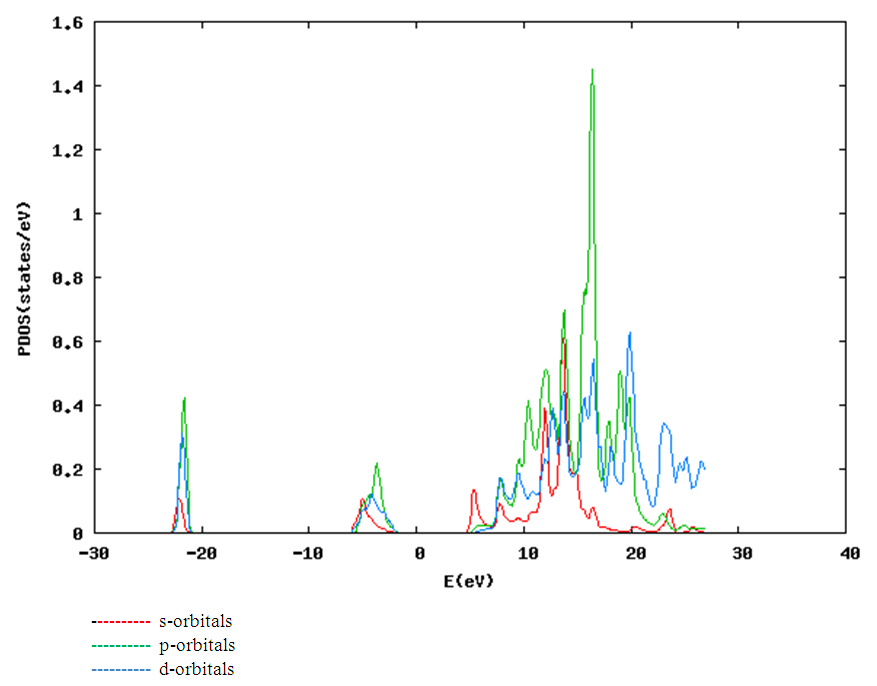Figure 11. Partial density of states (PDOS) of Magnesium (Mg)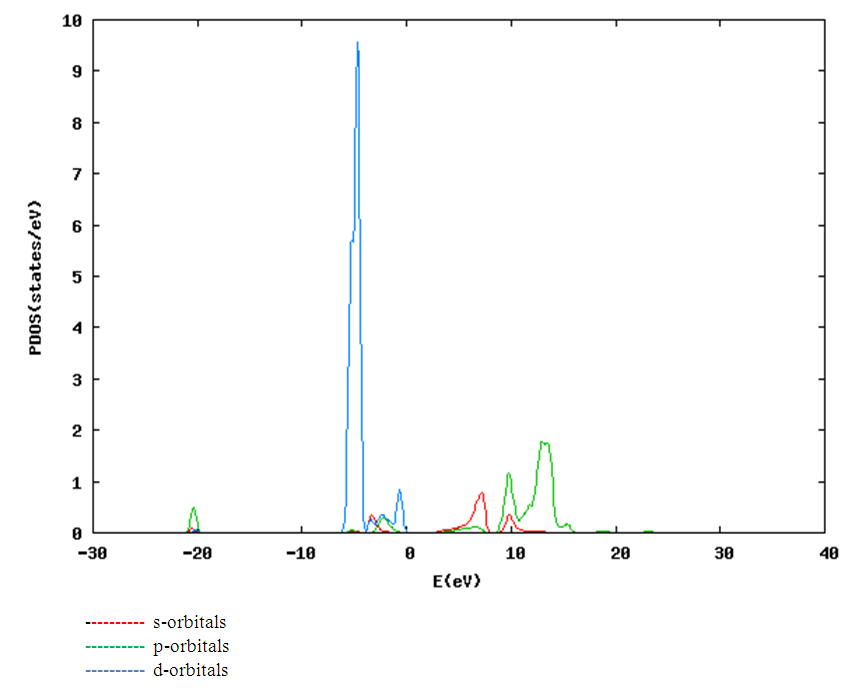Figure 12. Partial density of states (PDOS) of Cadmium (Cd)
Figure 13 presents the total DOS for the ternary alloy CaxCd1−xF2 for the concentration x = 0.25. There are five parts: the peak at the lowest energy states, is merely due to the s electrons of the fluorine, while the next part contains the contribution of Ca-s and Cd-s orbitals. The part just below the Fermi level is preponderantly p states of F. The p states of Ca and Cd dominate just above the fermi level. The next part contains the contribution of Cd-d orbitals.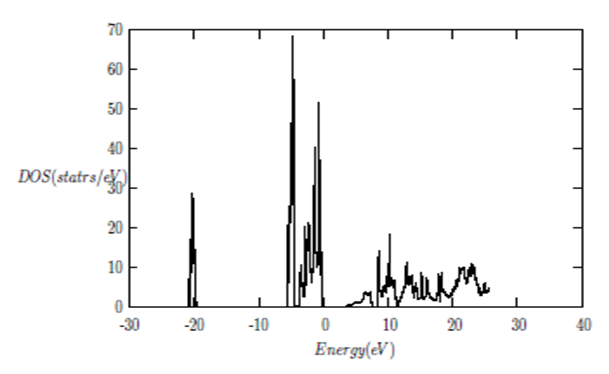Figure 13. Total density of states (DOS) of Ca0.25Cd0.75F2
Figures 14 and 15 show the total DOS for the ternary alloy CaxCd1−xF2 for the concentration x = 0.5 and 0.75. The two graphs are similar with different magnitudes of the peaks. There are six parts. The s electrons of the fluorine atoms dominate the part at the lowest energy states, while the next two parts contain the contribution of Ca-s and Cd-s orbitals respectively. The part just below the Fermi level is preponderantly p states of F with only a small contribution for p states of Ca and Cd. Just above the Fermi level the p states of Ca and Cd dominate. The next part contains the contribution of Cd-d orbitals.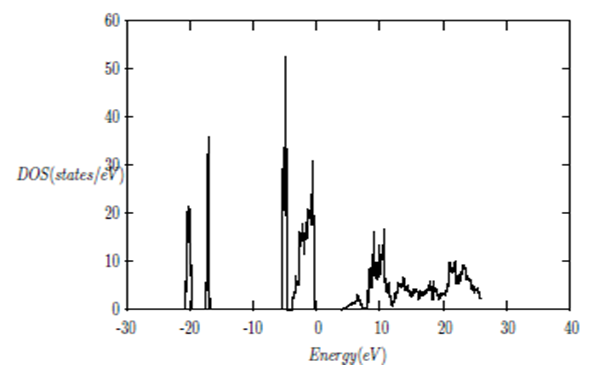Figure 14. Total density of states (DOS) of Ca0.50Cd0.50F2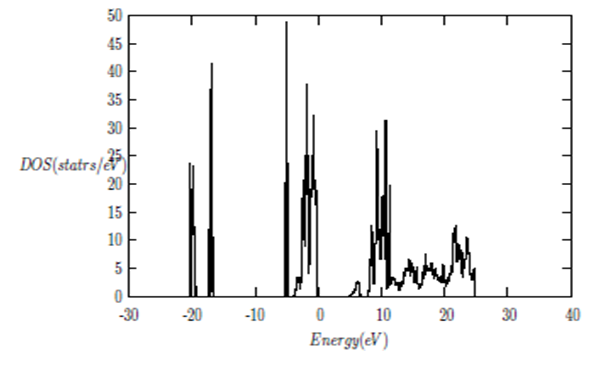Figure 15. Total density of states (DOS) of Ca0.75Cd0.25F2
The total density of states (DOS) for the ternary alloy CaxMg1−xF2 is shown in Figures 16, 17 and 18. The DOS for the concentration x = 0.25, 0.50 and 0.75 are very similar but the values of the peaks are different according to the concentration. There are four parts: the part at the lowest energy states is merely due to the s electrons of the fluorine, while the next part contains the contribution of Ca-s and Mg-s orbitals. The part just below the Fermi level EF is preponderantly p states of F. Just above the Fermi level the p states of Ca dominate.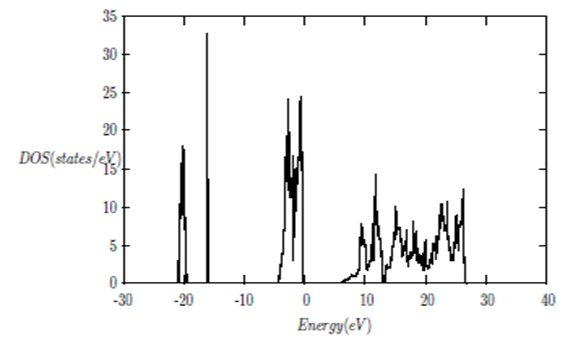Figure 16. Total density of states (DOS) of Ca0.25Mg0.75F2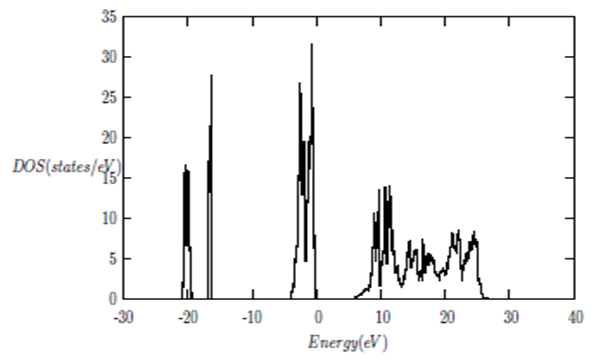Figure 17. Total density of states (DOS) of Ca0.50Mg0.50F2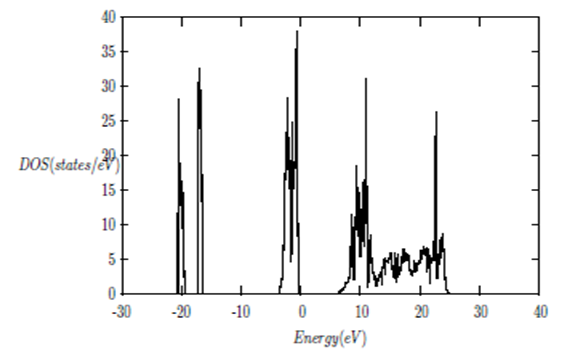Figure 18. Total density of states (DOS) of Ca0.75Mg0.25F2
Figure 12-18 show that as Ca composition increases in all the alloys, the density of states of Ca-s and Ca-p orbitals decrease while the density of states of Ca-d orbitals increase. The DOS of s orbital of F increase with Ca concentration in all the alloys while that of p orbitals decrease except in CaxMg1-xF2. The DOS of s, p and d orbitals of Cd and Mg decrease with Ca composition in their corresponding alloys.
Figure 19 shows the DOS for CaxCd1−xF2 at various concentration considered. One notices in Figure 17 that as the concentration of Cd decreases, the Fermi Level decreases linearly.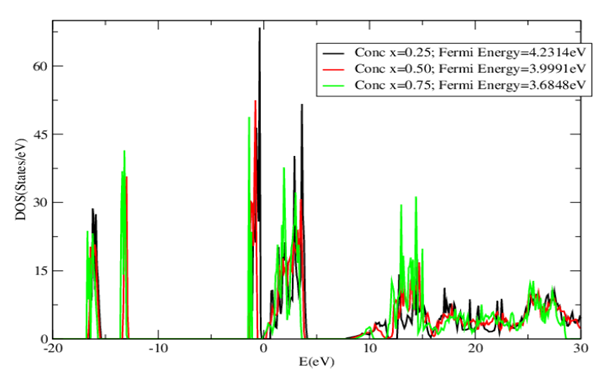Figure 19. The DOS of CaxCd1-xF2 showing decreasing Fermi Level as Cd decreases
Figure 20 shows the DOS for CaxMg1−xF2 at various concentration considered. There is non-linear relation between the concentration of Mg and Fermi Level.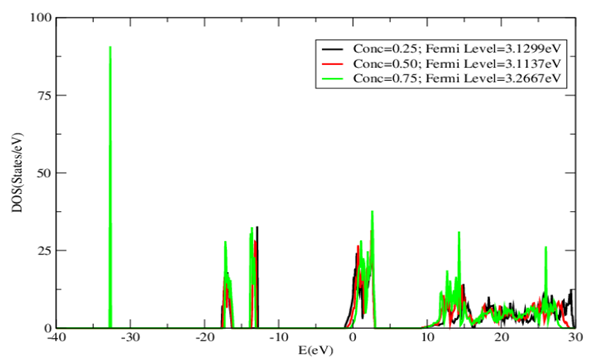Figure 20. The DOS of CaxMg1-xF2 at concentration x=0.25-0

### 4. Conclusions

First principles calculations as used in QUANTUM ESPRESSO (QE) were carried out to investigate the effects of concentration on band gap, structural and electronic properties of CaF2, CdF2, MgF2 and the ternary corresponding alloys CaxCd1-xF2 and CaxMg1-xF2 in cubic fluorite structure. We use the pseudopotential plane wave (PP-PW) method, in the framework of the density functional theory (DFT) with the Generalised Gradient Approximation (GGA). The lattice parameter and bulk modulus obtained for CaF2, CdF2 and MgF2 are in good agreement with experimental data. The lattice parameter of the CaxCd1-xF2 alloys increases with Ca concentration and the bulk modulus decreases with composition x. The band gaps are calculated using both PW-GGA and G0W0 methods. The alloys are insulators good for dielectrics. For ternary alloys CaxCd1−xF2 and CaxMg1−xF2, the valence band maximum and the conduction band minimum occur at the Γ point indicating that these ternary materials have direct band gaps. Lower energy states of the valence band are mainly due to the s electrons of F and cations while the upper energy states of the valence band are predominately p states of Fluorine. The p and d orbitals contribute majorly to the conduction band.